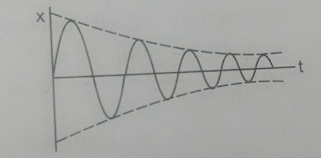Q

# How to solve this problem- A block of mass 0.1 kg is connected to an elastic spring of spring constant 640 Nm−1 and oscillates in a damping medium of damping constant 10−2 kg s−1. The system dissipates its energy gradually. The time

A block of mass 0.1 kg is connected to an elastic spring of spring constant 640 Nm−1 and oscillates in a damping medium of damping constant 102 kg s−1.  The system dissipates its energy gradually.  The time taken for its mechanical energy of vibration to drop to half of its initial value, is closest to :

• Option 1)

2 s

• Option 2)

3.5 s

• Option 3)

5 s

• Option 4)

7 s

1200 Views

As we learnt in

Resultant amplitude in damped oscillation -

- whereinSince the system dissipates its energy gradually and hence amplitude will also decrease with time

(i)

Energy of vibration drop to half of its initial value

from equation(i)

or

Correct option is 2.

Option 1)

2 s

This is an incorrect option.

Option 2)

3.5 s

This is the correct option.

Option 3)

5 s

This is an incorrect option.

Option 4)

7 s

This is an incorrect option.

Exams
Articles
Questions